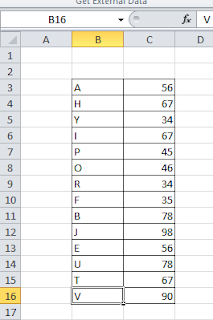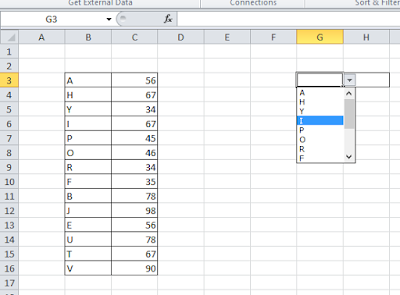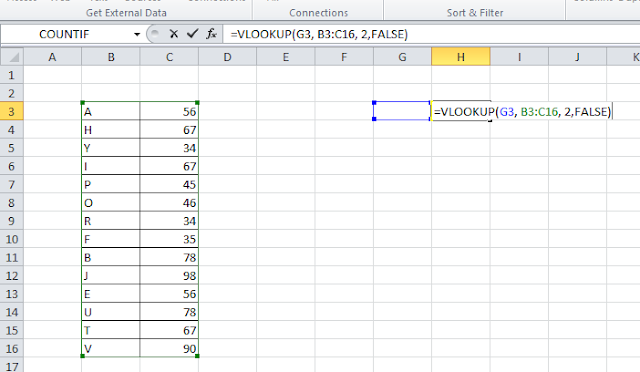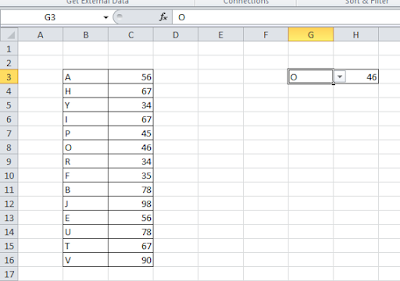# Lesson #9: VLOOKUP explained

In this post function VLOOKUP explained here once more with a very simple example.VLOOKUP looks for the value in the leftmost column of a table. It means the lookup_value column should be placed at the right side of the array and It will lookup for the value in leftmost columns of array.
I have made a simple table for giving an example of VLOOKUP. I have put alphabets in a column and in other column against those alphabets I have put some value. I will search the value against any alphabet with VLOOKUP.In a cell I put data validation from the list of alphabets for which I will look for value.In above picture I am showing how to use VLOOKUP. In the very next cell I have put the formula =VLOOKUP(G3, B3:C16, 2,FALSE). Structure of VLOOKUP is =VLOOKUP(lookup_value, table_array, col_index_num, [range_lookup]). This function will lookup for the value in that cell where I specified as lookup_value then It will search in the array for value in leftmost columns of the lookup_value column, then it will return the result.Here this function is showing result. I hope you like my post. Don’t forget to comment and share it.

Related video tutorials:Puspendu is the founder author of Excelabcd. He is a creative person, blogger and Excel-maniac guy.

Tagged with: